﻿ 实测海尖峰特性分析及抑制方法
«上一篇文章快速检索 高级检索

 雷达学报2015, Vol. 4Issue (3): 334-342  DOI: 10.12000/JR141080

### 引用本文 [复制中英文]

[复制中文]
Huang Yong, Chen Xiao-long, and Guan Jian. Property analysis and suppression method of real measured sea spikes[J] Journal of Radars, 2015, 4(3): 334-342. DOI: 10.12000/JR14108.
[复制英文]

### 文章历史

,,Property analysis and suppression method of real measured sea spikes
,,Department of Electronic and Information Engineering, Naval Aeronautical and Astronautical University, Yantai 264001, China
Abstract: In case of high-resolution, low grazing angle, high sea state, and horizontal transmitting, horizontal receiving polarization, the radar returns are strengthened, resulting in sea spikes. The sea spikes have the characteristics of high amplitudes, nonstationary, and non-Gaussian, which have a strong impact on the radar detection of weak marine moving targets. This study proposes a method for sea clutter suppression. Firstly, based on the sea spikes identification and selection method, the amplitude, temporal correlation, Doppler spectrum, and fractional power spectrum properties of sea spikes are analyzed. Secondly, the data to be detected are chosen by selecting the background clutter with minimum mean power, which can also eliminate the sea spikes. Correspondingly, sea clutter is suppressed with improved Signal-to-Clutter Ratio (SCR). Finally, the results of experiment with real radar data verify the effectiveness of the proposed method.
Key words: Sea clutter suppression     Sea spikes     Property analysis     Fractional power spectrum     Moving Target Detection (MTD)
1 引言

2 海尖峰判别和提取方法

 \left. \begin{align} & {{\left| x_{i}^{\text{HH}} \right|}^{2}}\ge T_{s}^{\text{HH}} \\ & W_{s}^{\text{HH}}\ge W_{\min }^{\text{HH}} \\ & I_{s}^{\text{HH}}\ge I_{\min }^{\text{HH}} \\ \end{align} \right\} (1)

 $T_{s}^{\text{HH}}=\frac{L}{N}\sum\limits_{i=1}^{N}{{{\left| x_{{}}^{\text{HH}}(i) \right|}^{2}}}$ (2)

3 海尖峰特性分析 3.1 幅值特性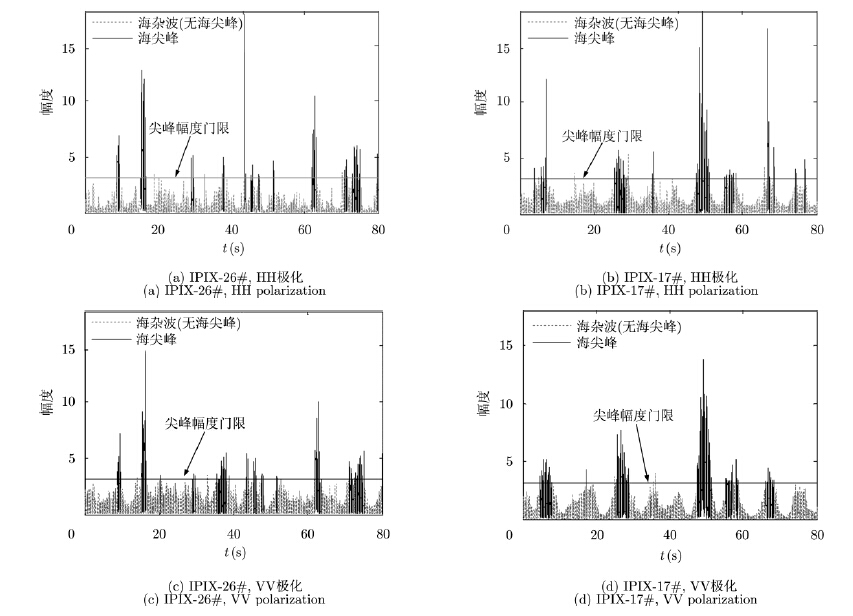图 1 海尖峰识别结果 Fig.1 Results of sea spikes identification表 1 IPIX数据描述 Tab. 1 Description of IPIX datasets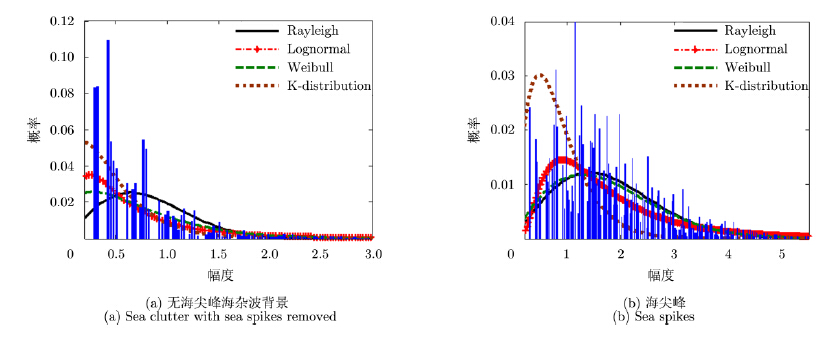图 2 海杂波时域幅度分布(IPIX-17#,HH极化) Fig.2 Amplitude distribution of sea spikes in time domain (IPIX-17#,HH polarization)表 2 海尖峰数量统计(%) Tab. 2 Quantity statistics of sea spikes (%)
3.2 时间相关性

 $\text{AC}{{\text{F}}_{n}}={\sum\limits_{i=1}^{N-1}{{{x}_{i}}x_{i+n}^{*}}}/{\sum\limits_{i=1}^{N-1}{{{x}_{i}}x_{i}^{*}}}\;$ (3)表 3 海尖峰相关时间统计 Tab. 3 ACF of sea spikes图 2 海杂波相关性(IPIX-17#) Fig.2 ACF property of sea clutter (IPIX-17#)
3.3 多普勒谱特性(频域特性)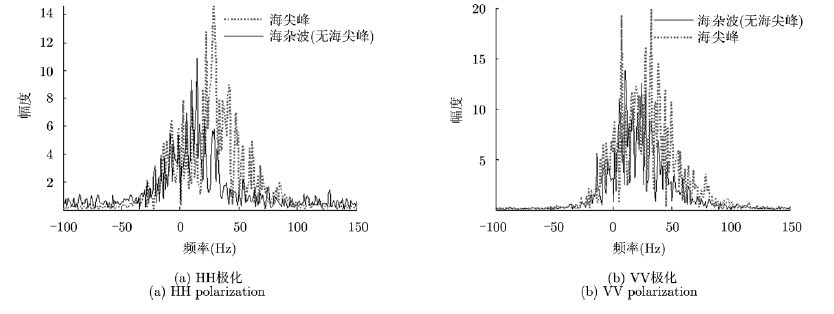图 4 海杂波多普勒谱(IPIX-17#,N=512) Fig.4 Doppler spectrum of sea clutter (IPIX-17#,N=512)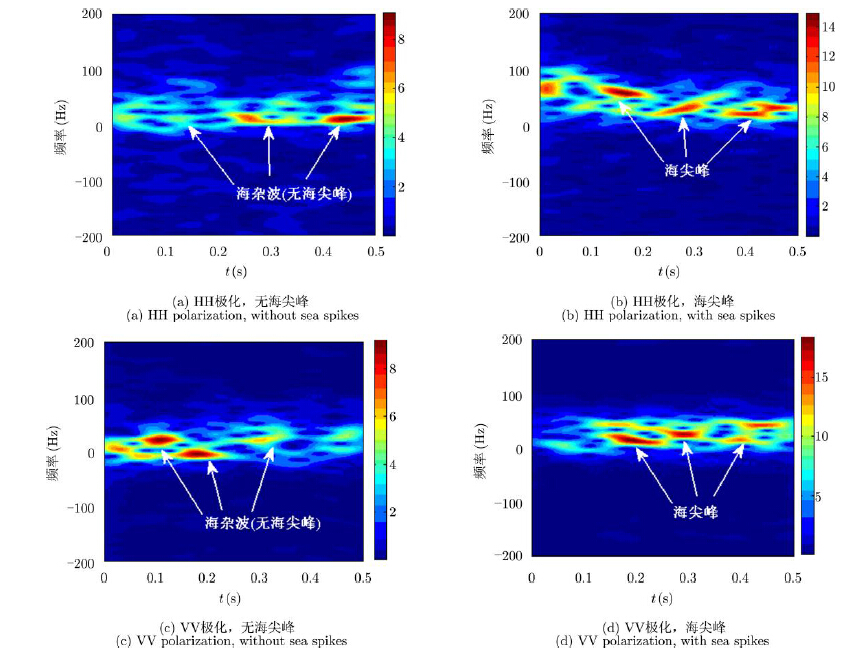图 5 海杂波STFT谱(IPIX-17#,N=512) Fig.5 STFT spectrum of sea clutter (IPIX-17#,N=512)
3.4 分数阶功率谱特性(分数阶傅里叶变换域特性)

 $P_{\xi }^{\alpha }(u)={{A}_{-\alpha }}{{F}_{\alpha }}[R_{\xi }^{\alpha }(\tau )](u){{\text{e}}^{-\text{j}\frac{{{u}^{2}}}{2}\cot \alpha }}$ (4)

 \begin{align} & R_{\xi }^{\alpha }(\tau )=\underset{T\to \infty }{\mathop{\lim }}\,\frac{1}{2T}\int_{-T}^{T}{{{R}_{\xi }}({{t}_{2}}+\tau ,{{t}_{2}}){{\text{e}}^{\text{j}{{t}_{2}}\tau \cot \alpha }}\text{d}{{t}_{2}}} \\ & \qquad \ \ ={{\left\langle {{R}_{\xi }}({{t}_{2}}+\tau ,{{t}_{2}}){{\text{e}}^{\text{j}{{t}_{2}}\tau \cot \alpha }} \right\rangle }_{{{t}_{2}}}} \end{align} (5)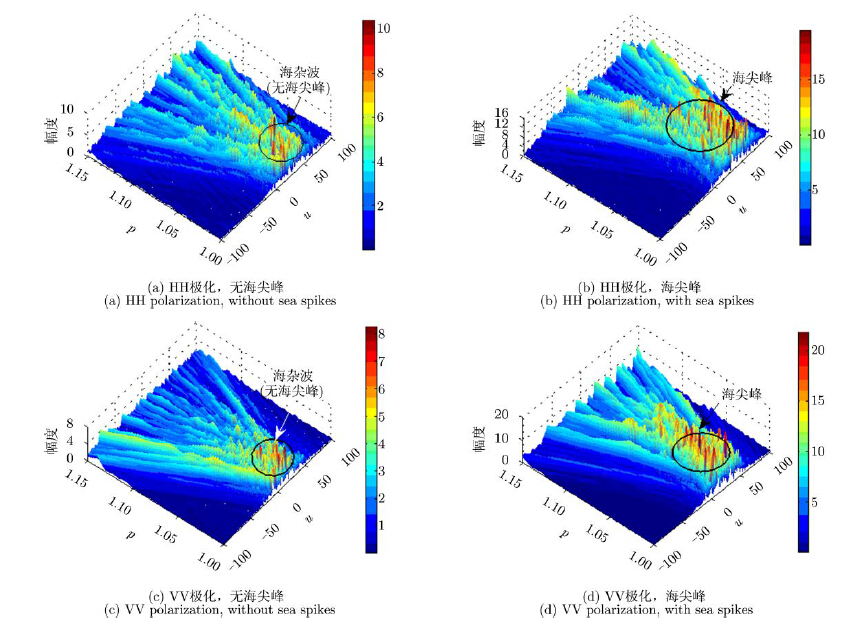图 6 海杂波分数阶功率谱(IPIX-17#,N=512) Fig.6 FRFT spectrum of sea clutter (IPIX-17#,N=512)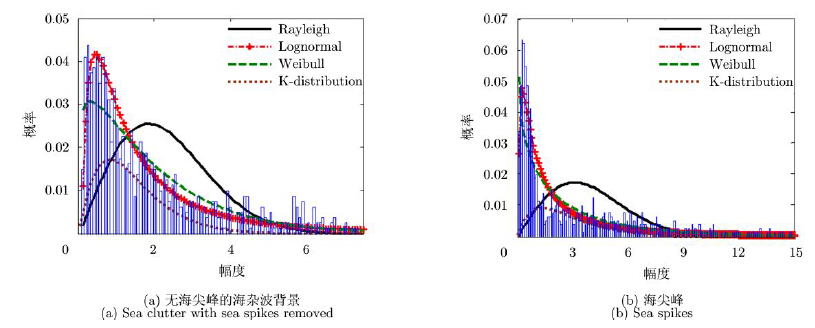图 7 海杂波FRFT域幅度分布(IPIX-17#,HH极化) Fig.7 Amplitude distribution of sea clutter in FRFT domain (IPIX-17#,HH polarization)
4 基于海尖峰判别和筛选的海杂波抑制方法

 \begin{align} & {{i}_{0}}=\underset{i}{\mathop{\arg \min }}\,\left( \frac{1}{{{M}_{i}}}\sum\limits_{j=1}^{{{M}_{i}}}{{{\left| {{x}_{i}}(j) \right|}^{2}}} \right),\ \\ & \qquad \qquad i=1,\ 2,\ \cdots ,\ n,\ j=1,\ 2,\cdots ,\ {{M}_{i}} \\ \end{align} (6)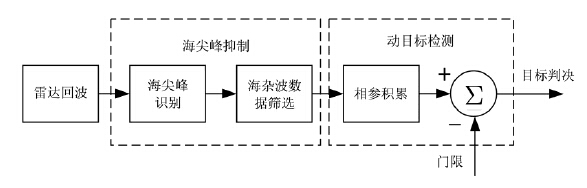图 8 海杂波抑制及动目标检测系统框图 Fig.8 Flowchart of sea clutter suppression and moving target detection
5 实测数据验证与分析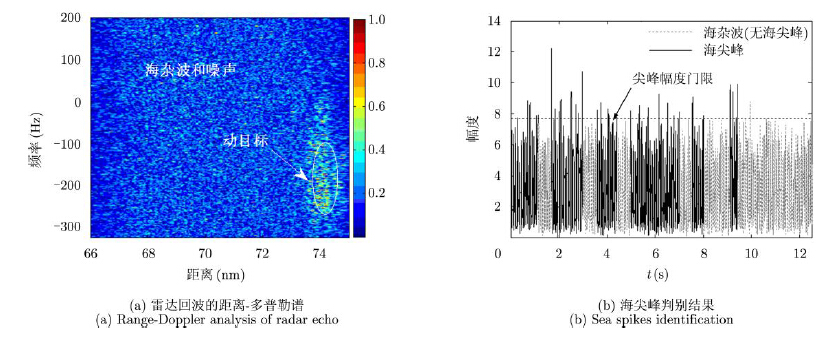图 9 雷达回波时频分析 Fig.9 Time-frequency analysis radar returns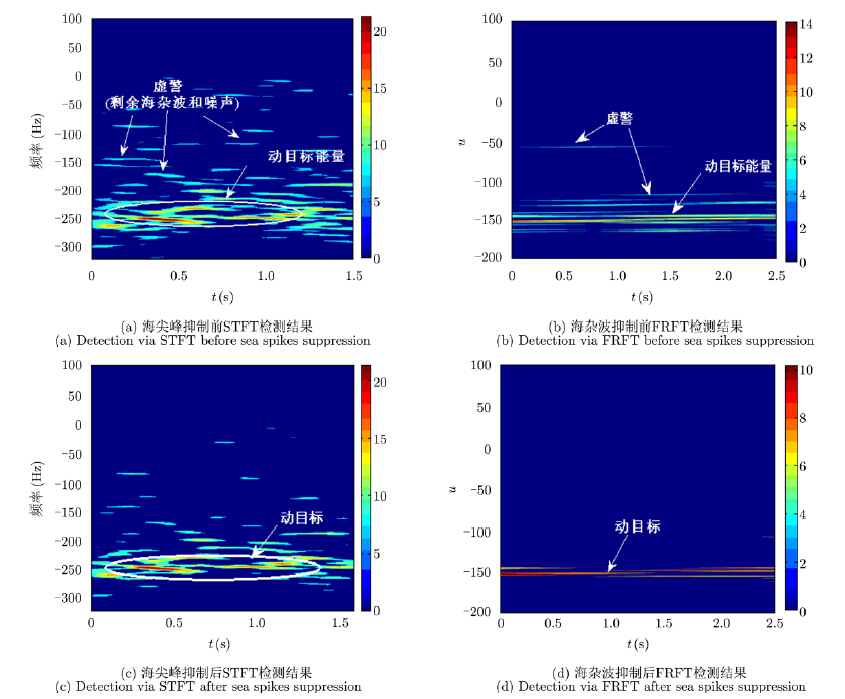图 10 海面动目标检测结果(${{P}_{\text{fa}}}\text{=1}{{\text{0}}^{-\text{4}}},$ N=1024) Fig.10 Detection results of sea surface moving target (${{P}_{\text{fa}}}\text{=1}{{\text{0}}^{-\text{4}}},$ N=1024)
6 结束语

  Zuo L, Li M, Zhang X W, et al.. An efficient method for detecting slow-moving weak targets in sea clutter based on time-frequency iteration decomposition[J]. IEEE Transactions on Geoscience and Remote Sensing, 2013, 51(6): 3659-3672.(1)  Chen X L, Guan J, Liu N B, et al.. Detection of a low observable sea-surface target with micromotion via the Radon-linear canonical transform[J]. IEEE Geoscience and Remote Sensing Letters, 2014, 11(7): 1225-1229.(1)  Ward K D and Watts S. Use of sea clutter models in radar design and development[J]. IET Radar, Sonar & Navigation, 2010, 4(2): 146-157.(1)  闫亮, 孙培林, 易磊, 等. 基于逆高斯分布的复合高斯海杂波建模研究[J]. 雷达学报, 2013, 2(4): 461-465. Yan L, Sun P L, Yi L, et al.. Modeling of compound-Gaussian sea clutter based on an inverse Gaussian distribution[J]. Journal of Radars, 2013, 2(4): 461-465.(1)  Zhang M, Chen H, and Yin H C. Facet-based investigation on EM scattering from electrically large sea surface with two-scale profiles: theoretical model[J]. IEEE Transactions on Geoscience and Remote Sensing, 2011, 49(6): 1967-1975.(1)  Greco M, Stinco P, and Gini F. Identification and analysis of sea radar clutter spikes[J]. IET Radar, Sonar & Navigation, 2010, 4(2): 239-250.(3)  陈小龙, 关键, 何友. 微多普勒理论在海面目标检测中的应用及展望[J]. 雷达学报, 2013, 2(1): 123-134. Chen X L, Guan J, and He Y. Applications and prospect of micro-motion theory in the detection of sea surface target[J]. Journal of Radars, 2013, 2(1): 123-134.(2)  Gutnik V G, Kulemin G P, and Sharapov L I. Spike statistics features of the radar sea clutter in the millimeter wave band at extremely small grazing angles[C]. The Fourth International Kharkov Symposium on Physics and Engineering of Millimeter and Sub-Millimeter Waves, Kharkov, Ukraine, 2001: 426-428.(1)  Posner F L. Spiky sea clutter at high range resolutions and very low grazing angles[J]. IEEE Transactions on Aerospace and Electronic Systems, 2002, 38(1): 58-73.(2)  梅孝安. IPIX雷达海尖峰统计特性研究[J]. 飞行器测控学报, 2007, 26(2): 19-23. Mei X A. A study on the statistical characteristics of IPIX radar sea spikes[J]. Journal of Spacecraft TT & C Technology, 2007, 26(2): 19-23.(1)  谢常清, 杨俊岭. 雷达海杂波尖峰时频分布特性研究[J]. 现代雷达, 2008, 30(5): 10-13. Xie C Q and Yang J L. A study on the time-frequency distribution of radar sea clutter spike[J]. Modern Radar, 2008, 30(5): 10-13.(1)  Rosenberg L. Sea-spike detection in high grazing angle X-band sea-clutter[J]. IEEE Transactions on Geoscience and Remote Sensing, 2013, 51(8): 4556-4562.(1)  Tao R, Zhang F, and Wang Y. Fractional power spectrum[J]. IEEE Transactions on Signal Processing, 2008, 56(9): 4199-4206.(2)  Drosopoulos A. Description of the OHGR database[R]. Ottawa: DREO Technical Note, 1994.(1)  Dong Y and Merrett D. Analysis of L-band multi-channel sea clutter[J]. IET Radar, Sonar & Navigation, 2010, 4(2): 223-238.(1)  Walker D. Doppler modelling of radar sea clutter[J]. IEE Proceedings-Radar, Sonar and Navigation, 2001, 148(2): 73-80.(1)  Chen X L, Guan J, Huang Y, et al.. Maneuvering target detection via Radon-fractional Fourier transform-based long- time coherent integration[J]. IEEE Transactions on Signal Processing, 2014, 62(4): 939-953.(1)  Chen X L, Guan J, Huang Y, et al.. Radon-linear canonical ambiguity function-based detection and estimation method for marine target with micromotion[J]. IEEE Transactions on Geoscience and Remote Sensing, 2015, 53(4): 2225-2240.(1)  Guida M, Longo M, and Lops M. Biparametric CFAR procedures for lognormal clutter[J]. IEEE Transactions on Aerospace and Electronic Systems, 1993, 29(3): 798-809.(1)